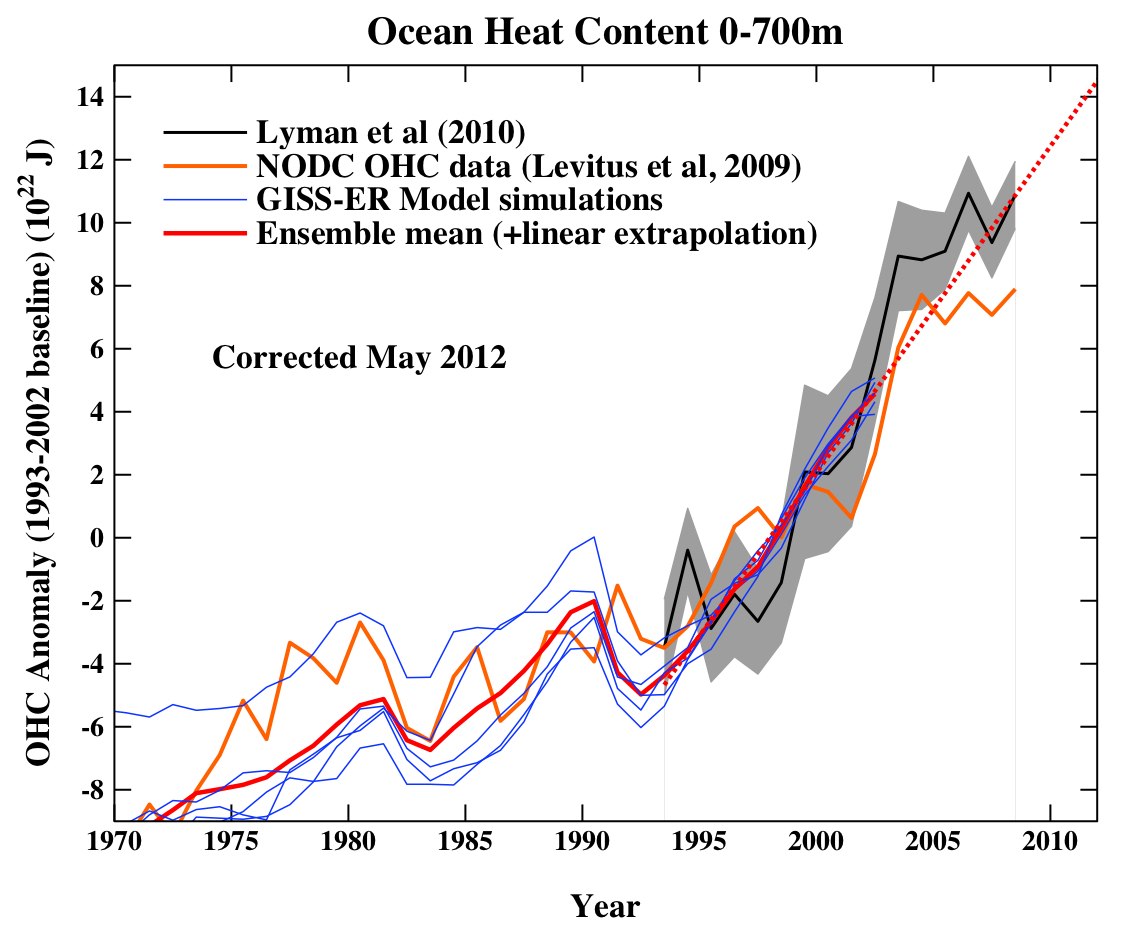## Friday, May 28, 2010

### Ocean heat content, and useful unitsThe recent post at realclimate about measured increases in ocean heat content has an interesting graph whose y-axis is labelled in spectacular units, 1022 J. Even exajoules are not that big! (1EJ = 1018 J)...
One comment on that blog suggested it would be good to re-express in other units that are more familiar - say degrees Celsius, or watts per square metre.
Here goes...
The graph shows the ocean heat content increasing by about 20 x 1022 J in 40 years.
First, let's express the change in heat content as a average rise in temperature of the top 700 metres of the ocean (which is what was actually measured to make these graphs!).
`Temperature rise = (Heat content increase) / (Volume of water) /                         (Heat capacity)                 = 20 x 1022 J /                    (350 x 106 km2 * 700 m) /                    (4.2 x 106 J/K/m3)                 = 0.19 K (or 0.19 degrees C).`

Second, let's express the rate of increase in heat content in terms of a net power per unit area required.
`Power per unit area = (Heat content increase) / Time / Area                    = 20 x 1022 J / (40 years) /                       (350 x 106 km2)                    = 0.45 W/m2.`

This can be compared with other things measured in the same units - see for example pages 20 and 170 in Sustainable Energy – without the hot air.
Hope this helps!

#### 3 comments:

Gavin McP said...

Loosely related to the post in question (communicating energy), I just came across this presentation from a US engineer on the energy implications of a low carbon lifestyle.

http://www.slideshare.net/energyliteracy/longnow-16-jan-09

Optimistic, but daunting.

Stephen Stretton said...

I really like this post, and think that ocean heat should give us a better estimate about the total radiative balance, and especially the 'fast feedbacks'.

For the period between 1995 and 2010:
Ocean heat content goes up by 10*10^22J.
Power per unit area = (Heat content increase) / Time / Area
= 1 x 10^23 J / (15 years*31 million seconds/yr) / (3.50 x 10^14 m2)
= (1 x 10^23 / 4.5*10^8) / 3.5 *10^14
=10/(4.5*3.5)
=0.625W/m2 over the oceans.

I just did the calculation because i think it is a good estimate of how much warming is actually happening.
I was going to compare this with Radiative Forcing, but they are not directly comparable of course, because of the possible 'fast feedbacks' such as water vapour and clouds, and the increase in outgoing radiation for a warmer earth.

The paper from a couple of years ago is interesting on this, and does this properly:
An observationally based energy balance for the Earth since 1950. D. M. Murphy,1 S. Solomon,1 R. W. Portmann,1 K. H. Rosenlof,1 P. M. Forster,2
and T. Wong3

However, I'm not so sure that the estimates of fast feedback are that certain.

It seems that we have an identity relation but there are two or three terms that are uncertain (fast feedback strength; aerosol; and possibly heat stored in deep ocean). If we only had one unknown term, we could use the heat flow identity to determine it from the other data, but with 2 or 3 its more difficult.

ismail said...

i have a small doubt about the unit conversion(for information). here heat content is expressed in W/m^2. how to convert this in terms of millimeter???